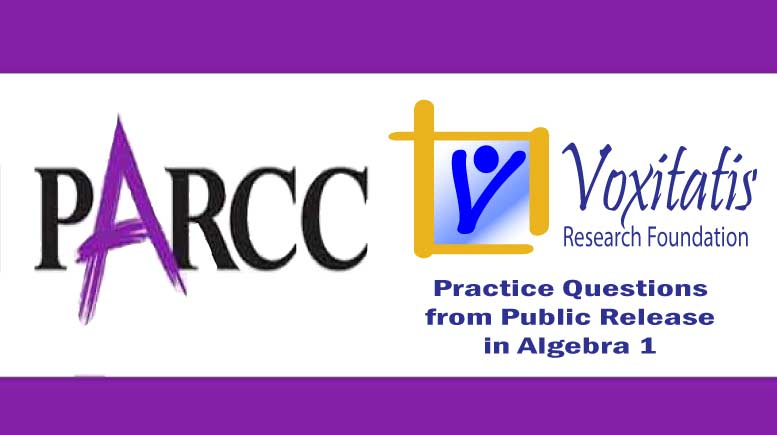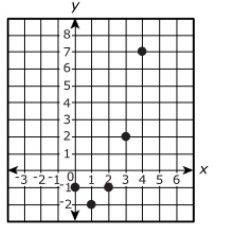Friday, September 17, 2021# Algebra 1 PARCC question: graph of function

-#### The following multiple-choice question, explained here in hopes of helping algebra students in Maryland and Illinois prepare for the PARCC test near the end of this school year, appears on the released version of PARCC’s Spring 2015 test in algebra 1, here:

Which is the graph of the function$y = (x-1)^2 - 2$ ?

• (A)• (B)• (C)• (D)PARCC evidence statement(s) tested: A-REI.10: Understand that the graph of an equation in two variables is the set of all its solutions plotted in the coordinate plane, often forming a curve (which could be a line).

The evidence statement above references Math Practice 7:

Mathematically proficient students look closely to discern a pattern or structure. Young students, for example, might notice that three and seven more is the same amount as seven and three more, or they may sort a collection of shapes according to how many sides the shapes have. Later, students will see 7 × 8 equals the well remembered 7 × 5 + 7 × 3, in preparation for learning about the distributive property. In the expression x2 + 9x + 14, older students can see the 14 as 2 × 7 and the 9 as 2 + 7. They recognize the significance of an existing line in a geometric figure and can use the strategy of drawing an auxiliary line for solving problems. They also can step back for an overview and shift perspective. They can see complicated things, such as some algebraic expressions, as single objects or as being composed of several objects. For example, they can see 5 – 3(x – y)2 as 5 minus a positive number times a square and use that to realize that its value cannot be more than 5 for any real numbers x and y.

The question tests students’ understanding of the Common Core high school algebra standard HSA.REI.D.10 (under Reasoning with Equations and Inequalities and Represent and solve equations and inequalities graphically), which states that students should “understand that the graph of an equation in two variables is the set of all its solutions plotted in the coordinate plane, often forming a curve (which could be a line).”

Solution strategy (there are others)

Recognize the domain of the function and pick the correct representation.

On inspection, the four options to this multiple choice question differ in two important aspects: (A) and (B) are scatter plots, and (C) and (D) are curves. Since x could equal 1.5 in the function given, (A) and (B) are ruled out—there’s no point above x = 1.5 on either (A) or (B).

That leaves (C) and (D). The second difference between these two is that (C) only goes between x = –1 and x = 3. Can x be 10 in this function?$y = ((10)-1)^2 - 2$$= 9^2 - 2$$= 81 - 2 = 79$

Sure, x can be 10, and the function would still be defined. Since the graph for (C) does not allow for x = 10 and the graph for (D) does, given the arrows, option (D) is the only possible correct answer.

## Resources for further study

Purple Math, developed by Elizabeth Stapel, a math teacher from the St Louis area, has a four-part series on graphing quadratic functions on a coordinate plane. She explains that, while you may start your graph by plotting points at integer x values, you have to finish the graph by drawing a smooth curve in between the points so that all points in the function’s domain, including those between the integers, are represented. The series starts here.

The Khan Academy, developed by Sal Khan, an engineer who has created a library of thousands of video lessons, has a few that demonstrate how to graph quadratic function (parabolas) on the coordinate plane. The series starts here, with “Intro to Parabolas.”

Chapter 5, Section 5-2 of Paul A Foerster’s book Algebra and Trigonometry deals with quadratic function graphs. He He advises, “the vertex is the most important point on the graph of a quadratic function. If you know where the vertex is, you can sketch a reasonably good parabola with very little other information.”

Complete reference: Foerster, Paul A. Algebra and Trigonometry: Functions and Applications, revised edition. Addison-Wesley, 1980, 1984. The book is used in several algebra classes taught in middle and high schools in both Illinois and Maryland.

## Analysis of this question and online accessibility

The question measures knowledge of the Common Core standard it purports to measure and tests students’ ability to recognize that the graph of a function in the coordinate plane includes all points in the domain, even those that fall in between the grid lines on the graph. It is considered to have a low cognitive demand.

The question can be tested online and should yield results that are as valid and reliable as those obtained on paper. The multiple-choice format may promote guessing, which casts doubt on the validity of the question.

No special accommodation challenges can be identified with this question, so the question is considered fair.

## Challenge

How would you define a function that has a domain that looks like (A), (B), or (C)? Can you identify a real-world situation that might have such a graph?

## Purpose of this series of posts

Voxitatis is developing blog posts that address every algebra 1 question released to the public by the Partnership for Assessment of Readiness for College and Careers, or PARCC, in order to help students prepare to take the test this spring.

Our total release will run from February 27 through March 15, with one or two questions discussed per day. Then we’ll move to geometry at the end of March, algebra 2 during the first half of April, and eighth grade during the last half of April.Paul Katulahttps://news.schoolsdo.org
Paul Katula is the executive editor of the Voxitatis Research Foundation, which publishes this blog. For more information, see the About page.

### Study finds increasing suicide rates among Black girls

0
Why are Black adolescent girls committing suicide at increasingly higher rates in the US? Could it be an effect of racism and prejudice?